# Object Tracking Using Time Difference of Arrival (TDOA)

This example shows how to track objects using time difference of arrival (TDOA). This example introduces the challenges of localization with TDOA measurements as well as algorithms and techniques that can be used for tracking single and multiple objects with TDOA techniques.

### Introduction

TDOA positioning is a passive technique to localize and track emitting objects by exploiting the difference of signal arrival times at multiple, spatially-separated receivers. Given a signal emission time (${\mathit{t}}_{\mathit{e}}$) from the object and the propagation speed ($\mathit{c}$) in the medium, the time-of-arrival (TOA) of the signal at 2 receivers located at ranges ${\mathit{r}}_{\text{\hspace{0.17em}}1}\text{\hspace{0.17em}}$and ${\mathit{r}}_{\text{\hspace{0.17em}}2}$ respectively from the object can be denoted as:

`$\begin{array}{l}{\mathit{t}}_{1\text{\hspace{0.17em}}}={\mathit{t}}_{\mathit{e}}+\frac{{\mathit{r}}_{1\text{\hspace{0.17em}}}}{\mathit{c}}\\ {\mathit{t}}_{2\text{\hspace{0.17em}}}={\mathit{t}}_{\mathit{e}}+\frac{{\mathit{r}}_{2}}{\mathit{c}}\end{array}$`

As the true signal emission time is typically not known, a difference between ${\mathit{t}}_{1\text{\hspace{0.17em}}}$and ${\mathit{t}}_{2\text{\hspace{0.17em}}}$can be used to obtain some information about the location of an object. Specifically, given the location of each receiver, the time-difference measurement corresponds to a difference in ranges from the two receivers to the unknown object.

`$\sqrt{{\left({\mathit{x}}_{\mathit{t}}-{\mathit{x}}_{1}\right)}^{2\text{\hspace{0.17em}}}+{\left({\mathit{y}}_{\mathit{t}}-{\mathit{y}}_{1\text{\hspace{0.17em}}}\right)}^{2\text{\hspace{0.17em}}}{\text{\hspace{0.17em}}+\text{\hspace{0.17em}}\left({\mathit{z}}_{\mathit{t}}-{\mathit{z}}_{1\text{\hspace{0.17em}}}\right)}^{2}}-\sqrt{{\left({\mathit{x}}_{\mathit{t}}-{\mathit{x}}_{2}\right)}^{2\text{\hspace{0.17em}}}+{\left({\mathit{y}}_{\mathit{t}}-{\mathit{y}}_{2\text{\hspace{0.17em}}}\right)}^{2\text{\hspace{0.17em}}}{\text{\hspace{0.17em}}+\text{\hspace{0.17em}}\left({\mathit{z}}_{\mathit{t}}-{\mathit{z}}_{2\text{\hspace{0.17em}}}\right)}^{2}}=\mathit{c}\left({\mathit{t}}_{1}-{\mathit{t}}_{2\text{\hspace{0.17em}}}\right)$`

where $\left[{\mathit{x}}_{\mathit{t}}\text{\hspace{0.17em}}{\mathit{y}}_{\mathit{t}\text{\hspace{0.17em}}}\text{\hspace{0.17em}}{\mathit{z}}_{\mathit{t}\text{\hspace{0.17em}}}\right]$ is the unknown object location, ${\mathit{t}}_{1}-{\mathit{t}}_{2\text{\hspace{0.17em}}}$ is the TDOA measurement, and $\left[{\mathit{x}}_{1\text{\hspace{0.17em}}}{\mathit{y}}_{1}\text{\hspace{0.17em}}{\mathit{z}}_{1\text{\hspace{0.17em}}}\right]$, $\left[{\mathit{x}}_{2\text{\hspace{0.17em}}}{\mathit{y}}_{2}\text{\hspace{0.17em}}{\mathit{z}}_{2\text{\hspace{0.17em}}}\right]$ are the locations of two receivers. This non-linear relationship between TDOA and object location represents a hyperbola in 2-D or a hyperboloid in 3-D coordinates with two receivers at the foci. The image below shows the hyperbolae of an object for different TDOA measurements ($\mathit{c}$ = speed of light). These curves are typically called constant TDOA curves. Notice that using the sign of a TDOA measurement, you can constrain the object location to a single branch of the hyperbola.

`HelperTDOATrackingExampleDisplay.plotConstantTDOACurves;`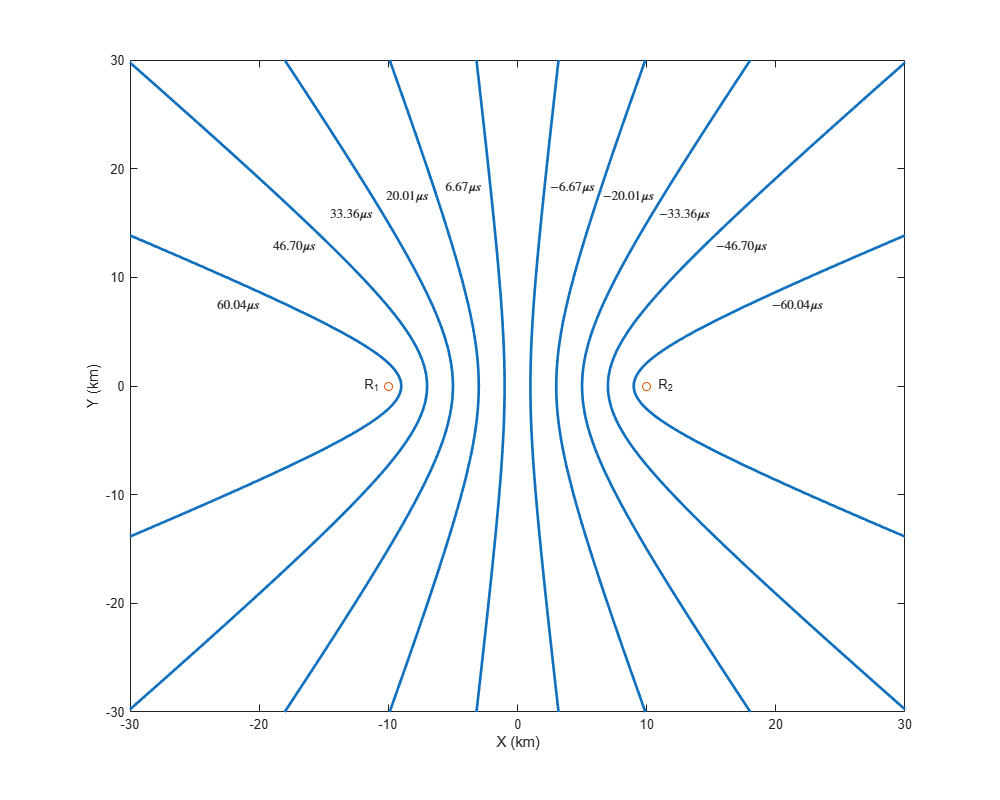#### TDOA Calculation

There are two primary methods used to calculate the TDOA measurement from the signal of an object. In the first method, each receiver measures the absolute time instant of signal arrival (time-of-arrival or TOA) as defined by ${\mathit{t}}_{\mathit{i}\text{\hspace{0.17em}}}$above. A difference in time-of-arrivals between two receivers is then calculated to obtain the TDOA measurement. This method is applicable when certain signal attributes are known a-priori and the leading edge of the signal can be detected by the receiver.

`${\mathrm{TDOA}}_{12\text{\hspace{0.17em}}}={\mathrm{TOA}}_{2\text{\hspace{0.17em}}}-{\mathrm{TOA}}_{1\text{\hspace{0.17em}}}$`

In the second method, each receiver records and transmits the received signal to a shared processing hub. A cross-correlation between signals received from two receivers is calculated at the processing hub. The TDOA is estimated to be the delay which maximizes the cross-correlation between the two signals.

`${\mathrm{TDOA}}_{12\text{\hspace{0.17em}}}=\underset{\mathit{t}\in \left[-{\mathit{T}}_{\mathrm{max}}\text{\hspace{0.17em}}{\mathit{T}}_{\mathrm{max}}\text{\hspace{0.17em}}\right]}{\mathrm{argmax}}\left(\left[{\mathit{S}}_{1\text{\hspace{0.17em}}}\star {\mathit{S}}_{2}\right]\left(\mathit{t}\right)\right)$`

where $\left[{\mathit{S}}_{1\text{\hspace{0.17em}}}\star {\mathit{S}}_{2}\right]\left(\mathit{t}\right)$ represents the cross-correlation between the signals at receivers as a function of time delay, $\mathit{t}$. ${\mathit{T}}_{\mathrm{max}}$ is the maximum possible absolute delay and can be calculated as $\frac{\mathit{D}}{\mathit{c}\text{\hspace{0.17em}}}$, where $\mathit{D}$ is the distance between the receivers.

Both methods of TDOA calculation require synchronized clocks at the receivers. In practical applications, you typically achieve this using the Global Positioning System (GPS) clock.

#### TDOA Localization

As the TDOA between two receivers localizes an object to a hyperbola or hyperboloid, it is not possible to observe the full state of the object by using only two stationary receivers. For 2-D localization, at least 3 spatially-separated receivers are required to estimate the object state. Similarly, for 3-D tracking, at least 4 spatially-separated receivers are required.

With $\mathit{N}\text{\hspace{0.17em}}\left(\mathit{N}\ge 2\right)$ receivers, a total of $\mathit{N}\left(\frac{\mathit{N}-1}{2\text{\hspace{0.17em}}}\right)$ TDOA measurements from an object can be obtained by calculating the time difference of arrival using each combination of receiver. However, out of these measurements, only $\mathit{N}-1\text{\hspace{0.17em}}$measurements are independent and the rest of the TDOA measurements can be formulated as a linear combination of these independent measurements. Given these $\mathit{N}-1$ measurements from the object, an estimation algorithm is typically used to locate the position of the object. In this example, you use the spherical intersection algorithm  to find the position and uncertainty in position estimate. The spherical intersection algorithm assumes that all $\mathit{N}-1$ TDOAs are calculated with respect to the same receiver, sometimes referred to as the reference receiver.

The accuracy or uncertainty in the estimated position obtained from localization depends on the geometry of the network. At regions, where small errors in TDOA measurements results in large errors in estimated position, the accuracy of the estimated position is lower. This effect is called geometric dilution of precision (GDOP). The image below shows the GDOP heat map of the network geometry for a 2-D scenario with 3 receivers. Note that the accuracy is high within the envelope generated by the network and is lowest directly behind the receivers along the receiver-to-receiver line of sights.

`HelperTDOATrackingExampleDisplay.plotGDOPAccuracyMap;`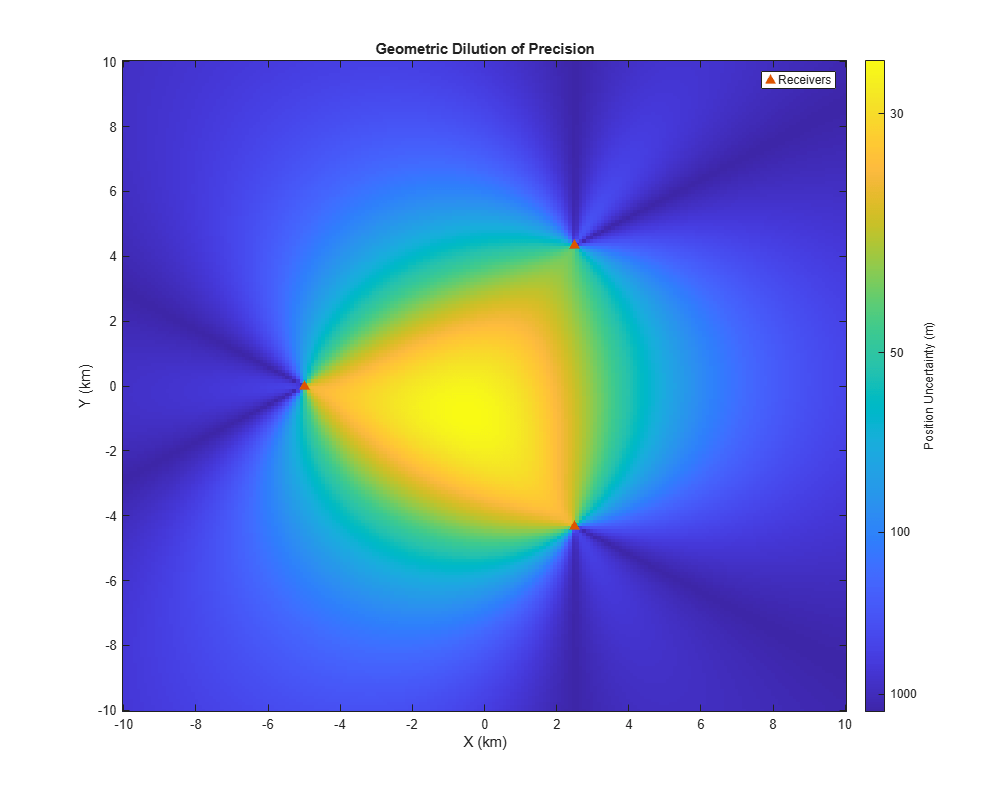### Tracking a Single Emitter

In this section, you simulate TDOA measurements from a single object and use them to localize and track the object in the absence of any false alarms and missed detections.

You define a 2-D scenario using the helper function, `helperCreateSingleTargetTDOAScenario`. This function returns a `trackingScenarioRecording` object representing a single object moving at a constant velocity. This object is observed by three stationary, spatially-separated receivers. The helper function also returns a matrix of two columns representing the pairs formed by the receivers. Each row represents the `PlatformID` of the platforms that form the TDOA measurement.

```% Reproducible results rng(2021); % Setup scenario with 3 receivers numReceivers = 3; [scenario, rxPairs] = helperCreateSingleTargetTDOAScenario(numReceivers);```

You then use the helper function, `helperSimulateTDOA,` to simulate TDOA measurements from the objects. The helper function allows you to specify the measurement accuracy in the TDOA. You use a measurement accuracy of 100 nanoseconds to represent timing inaccuracy in the clocks as well as TDOA estimation. The emission speed and units of the reported time are speed of light in vacuum and nanoseconds respectively, specified using the `helperGetGlobalParameters` function. The `helperSimulateTDOA` function outputs TDOA detections as a cell array of `objectDetection` objects.

```tdoaDets = helperSimulateTDOA(scenario, rxPairs, measNoise); % Specify measurement noise variance (nanosecond^2) ```

Each TDOA detection is represented using the `objectDetection` according to the following format:Next, you run the scenario and generate TDOA detections from the object. You use the helper function, `helperTDOA2Pos`, to estimate the position and position covariance of the object at every step using the spherical intersection algorithm . You further filter the position estimate of the object using a global nearest neighbor (GNN) tracker configured using the `trackerGNN` System object™ with a constant-velocity linear Kalman filter. To account for the high speed of the object, this constant velocity Kalman filter is configured using the helper function, `helperInitHighSpeedKF`.

```% Define accuracy in measurements measNoise = (100)^2; % nanoseconds^2 % Display object display = HelperTDOATrackingExampleDisplay(XLimits=[-1e4 1e4],... YLimits=[-1e4 1e4],... LogAccuracy=true,... Title="Single Object Tracking"); % Create a GNN tracker tracker = trackerGNN(FilterInitializationFcn=@helperInitHighSpeedKF,... AssignmentThreshold=100); while advance(scenario) % Elapsed time time = scenario.SimulationTime; % Simulate TDOA detections without false alarms and missed detections tdoaDets = helperSimulateTDOA(scenario, rxPairs, measNoise); % Get estimated position and position covariance as objectDetection % objects posDet = helperTDOA2Pos(tdoaDets,true); % Update the tracker with position detections tracks = tracker(posDet, time); % Display results display(scenario, rxPairs, tdoaDets, {posDet}, tracks); end```

Notice that the tracker is able to maintain a track on the object. The the estimated position of the object lies close to the intersection of hyperbolic curves created by each receiver pair. Also notice in the zoomed plot below that the filtered estimate of the object has less uncertainty as compared to the fused estimate at every step. As the object moves towards positive X axis, the error in estimated position increases due to geometric dilution of precision. Notice that the tracker is able to provide an improved estimate of the object by filtering the position estimate of the object using the Kalman filter with constant velocity motion model.

`zoomOnTrack(display,tracks(1).TrackID);`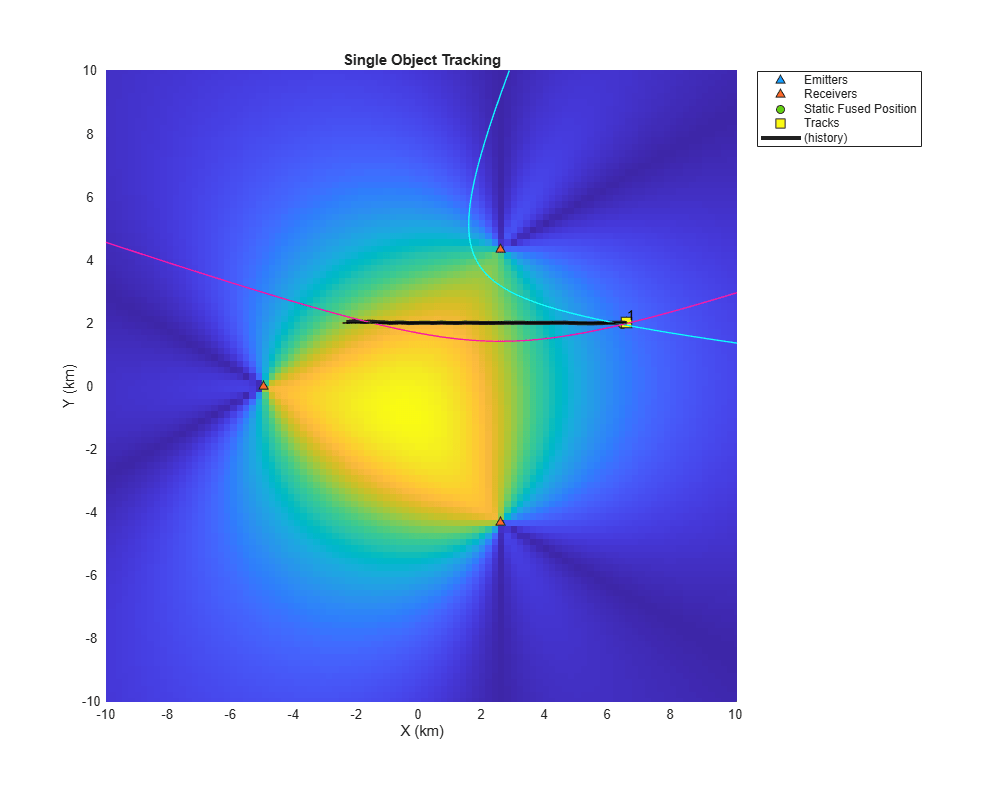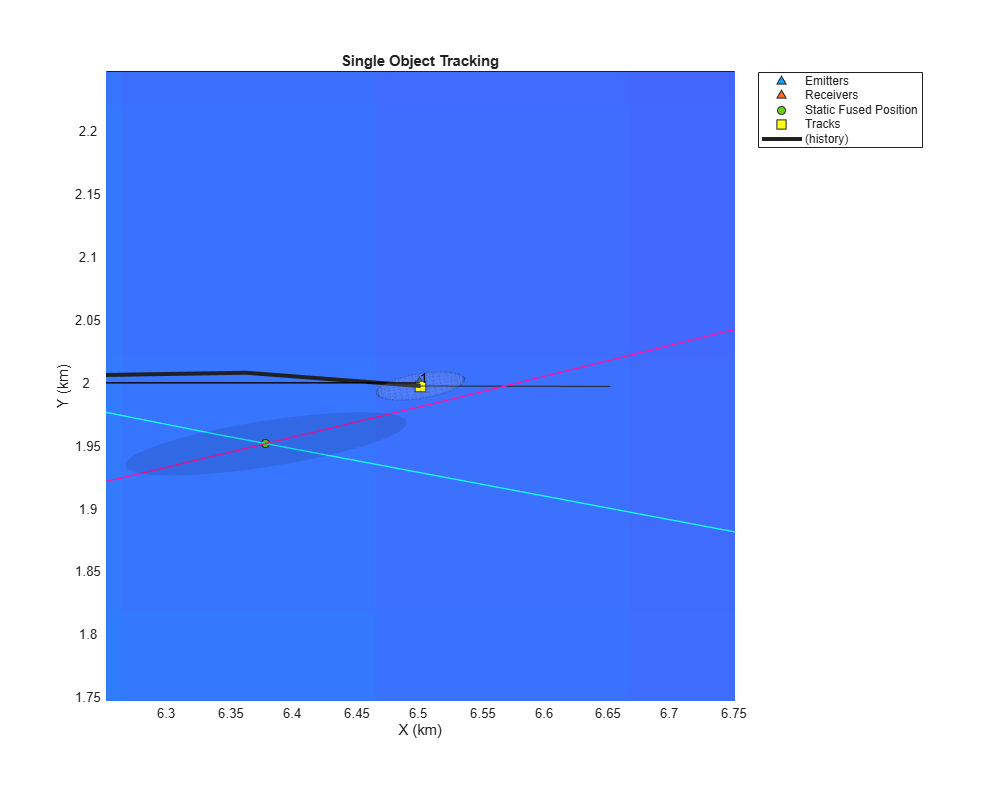`plotDetectionVsTrackAccuracy(display);`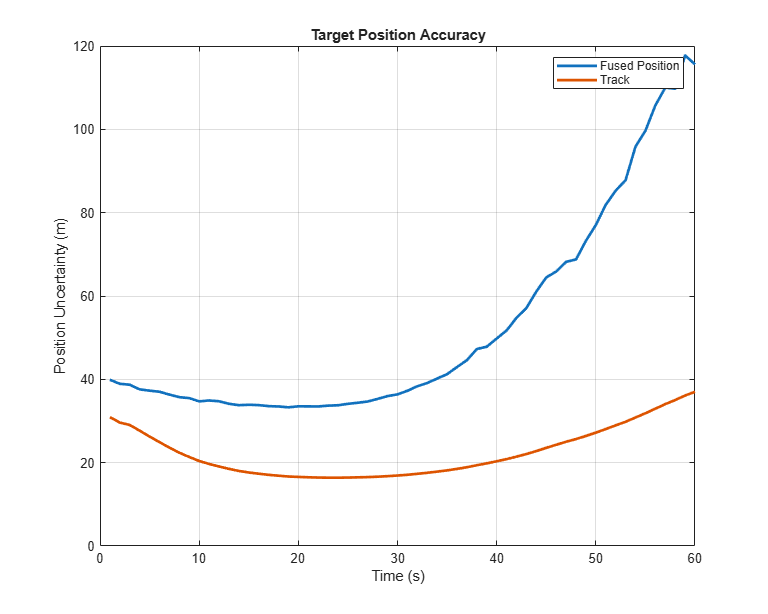### Tracking Multiple Emitters with Known IDs

In the previous section, you learned how to use TDOA measurements from a single object to generate positional measurement of the object. In practical situations, the scenario may consist of multiple objects.

The main challenge in multi-object TDOA tracking is the estimation of TDOA for each target. In the presence of multiple objects, each receiver receives signals from multiple objects. In the first method for TDOA calculation, each receiver records multiple time-of-arrival measurements. The unknown data association between time-of-arrival measurements reported by each receiver results in many possible TDOA combinations. In the second method of TDOA calculation, the signal cross-correlation function between receiver signals results in multiple peaks corresponding to true objects as well as false alarms. In certain applications, this unknown data association between receivers can be easily solved at the signal level. For example, if the signal encoding is known a-priori, such as in wireless communications signals like LTE, 5G signals or aviation communication signals like ADSB signals, the receiver is typically able to calculate both the time-of-arrival and the unique identity of the object. Similarly, if the objects are separated in their carrier frequencies, a separate TDOA calculation can be added for each object in their own frequency bands.

In this section, you assume that signal-level data association is performed at the receiver. This helps the processing hub to form TDOA measurements per identified object without ambiguity. To simulate TDOA measurements with known emitter identification, you provide a fourth input argument to the helper function, `helperSimulateTDOA`. The function outputs the unique identity of the emitter as the `objectClassID` property of the `objectDetection` object. You use this object identity, shared between TDOAs from multiple receiver-pairs, to fuse detections from each object separately and obtain their respective positions as well as uncertainties. Then, you use these fused measurements to track these objects using the GNN tracker.

```% Create the multiple object scenario numReceivers = 3; numObjects = 4; [scenario, rxPairs] = helperCreateMultiTargetTDOAScenario(numReceivers, numObjects); % Reset display release(display); display.LogAccuracy = false; display.Title = 'Tracking Multiple Emitters with Known IDs'; % Release tracker release(tracker); while advance(scenario) % Elapsed time time = scenario.SimulationTime; % Simulate classified TDOA detections classifiedTDOADets = helperSimulateTDOA(scenario, rxPairs, measNoise, true); % Find unique object identities tgtIDs = cellfun(@(x)x.ObjectClassID,classifiedTDOADets); uniqueIDs = unique(tgtIDs); % Estimate the position and covariance of each emitter as objectDection posDets = cell(numel(uniqueIDs),1); for i = 1:numel(uniqueIDs) thisEmitterTDOADets = classifiedTDOADets(tgtIDs == uniqueIDs(i)); posDets{i} = helperTDOA2Pos(thisEmitterTDOADets, true); end % Update tracker tracks = tracker(posDets, time); % Update display display(scenario, rxPairs, classifiedTDOADets, posDets, tracks); end```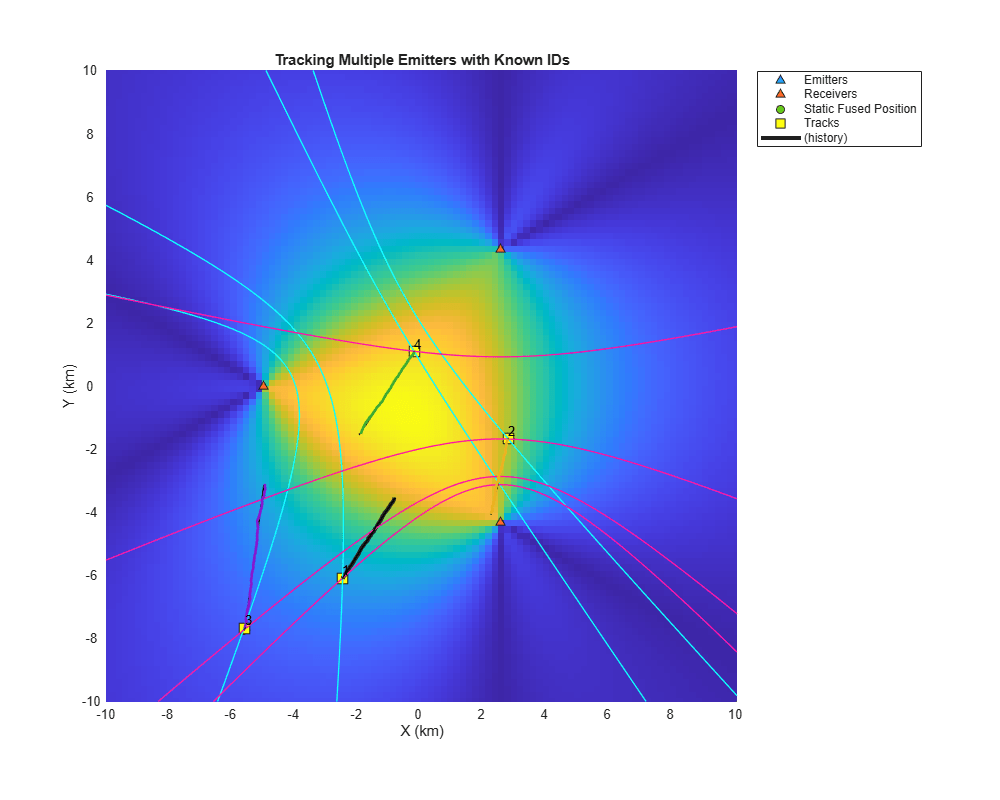Note that the tracker is able to maintain a track on all 4 objects when TDOA-to-TDOA association is assumed to be known. When the data association is provided by the receivers, the multi-object TDOA estimation is simplified to generating multiple TDOA detections with known single-object associations. The multi-object tracking problem is similarly simplified because there are no false TDOA detections.

### Tracking Multiple Emitters with Unknown IDs

In this section, you assume that data association between receiver data is not known a-priori i.e., receivers cannot identify objects. In the absence of this data association, each intersection between blue and maroon hyperbolic curves shown in the plot from the Tracking Multiple Emitters with Known IDs section could be a possible object location. Therefore, when data association is not available from the receivers, associating time-of-arrival (TOA) or TDOA detections from multiple receivers is prone to detections from ghost objects. To separate the ghost detections from true object detections, you must add more receivers to reduce the ambiguity. In this section, you will use a static fusion algorithm  to determine the unknown data association and estimate position measurements from each object. You will use this static fusion algorithm for systems that transmit multiple time-of-arrival measurements to the processing hub as well as systems that transmit recorded signals to the hub and calculates TDOA before processing them for object tracking.

#### Tracking with Time-of-Arrival (TOA) Measurements

In this section, you configure a system in which each receiver transmits multiple time of arrival (TOA) measurements to the central processing hub. To reduce ambiguity in data association and to reduce the number of potential ghost objects, you use 4 stationary receivers to localize and track the objects.

To simulate time-of-arrival measurements from the objects, you use the helper function, `helperSimulateTOA`, to simulate time-of-arrival measurements from multiple objects. These time-of-arrival measurements record the exact time instant in a global reference clock at which the signal was received by the receivers. To simulate exact time instants, the helper function uses the scenario time as the global clock time and assumes that the objects emit signals at the time instant of scenario. Note that the algorithm used to track with these measurements is not tied to this assumption as the exact emission time from the object is not known to the receivers.

You specify the `nFalse` input as 1 to simulate one false time-of-arrival measurement from each receiver. You also specify the `Pd` input, which defines the probability of detecting a true time-of-arrival measurement by each receiver, as 0.95.

```toaDets = helperSimulateTOA(scenario, receiverIDs, measNoise, nFalse, Pd); % receiverIDs - PlatformID of all receivers % measNoise - Accuracy in TOA measurements (ns^2) % nFalse - number of false alarms per receiver % Pd - Detection probability for receivers ```

Here is the format of time-of-arrival (TOA) detections.To fuse multiple time-of-arrival measurements from each receiver, you use the static fusion algorithm by configuring a `staticDetectionFuser` object. The `staticDetectionFuser` object determines the best data association between time of arrival measurements and provides fused detections from possible objects. The static fusion algorithm requires two sub routines or functions. The first function (`MeasurementFusionFcn`) allows the algorithm to estimate a fused measurement from object, given a set of time-of-arrival measurements assumed to originate from the same object. The second function (`MeasurementFcn`) allows the algorithm to obtain the expected time-of-arrival measurement from the fused measurement. Note that to define a measurement function to obtain time-of-arrival measurement, the fused measurement must contain an estimate of the signal emission time from the object. Therefore, you define the fused measurement as $\left[{\mathit{x}}_{\mathit{t}}\text{\hspace{0.17em}}{\mathit{y}}_{\mathit{t}\text{\hspace{0.17em}}}\text{\hspace{0.17em}}{\mathit{z}}_{\mathit{t}}\text{\hspace{0.17em}}{\mathit{t}}_{\mathit{e}}\right]$, where ${\mathit{t}}_{\mathit{e}}$ is the time instant at which the object emitted the signal.

You use the helper function, `helperTOA2Pos`, to estimate fused measurement - position and emission time - of the object. You also use the helper function, `helperMeasureTOA`, to define the measurement model that calculates time-of-arrival measurement from the fused measurement. The fusion algorithm outputs fused detections as a cell array of `objectDetection` objects. Each element of the cell array defines an `objectDetection` object containing measurement from a potential object as its position and emission time. You can speed-up this static fusion algorithm by specifying the `UseParallel` property to `true`. Specifying `UseParallel` as `true` requires the Parallel Computing Toolbox™.

```% Create scenario [scenario, ~, receiverIDs] = helperCreateMultiTargetTDOAScenario(4, 4); % Specify stastics of TOA simulation measNoise = 1e4; % 100 ns per receiver nFalse = 1; % 1 false alarm per receiver per step Pd = 0.95; % Detection probability of true signal % Release display release(display); display.Title = 'Tracking Using TOA Measurements with Unknown IDs'; % Release tracker release(tracker); tracker.ConfirmationThreshold = [4 6]; % Use Parallel Computing Toolbox if available useParallel = false; % Define fuser. toaFuser = staticDetectionFuser(MaxNumSensors=4,... MeasurementFormat='custom',... MeasurementFcn=@helperMeasureTOA,... MeasurementFusionFcn=@helperTOA2Pos,... FalseAlarmRate=1e-8,... DetectionProbability=Pd,... UseParallel=useParallel); while advance(scenario) % Current time time = scenario.SimulationTime; % Simulate TOA detections with false alarms and missed detections toaDets = helperSimulateTOA(scenario, receiverIDs, measNoise, Pd, nFalse); % Fuse TOA detections to estimate position amd emission time of % unidentified and unknown number of emitters. [posDets, info] = toaFuser(toaDets); % Remove emission time before feeding detections to the tracker as it % is not tracked in this example. for i = 1:numel(posDets) posDets{i}.Measurement = posDets{i}.Measurement(1:3); posDets{i}.MeasurementNoise = posDets{i}.MeasurementNoise(1:3,1:3); end % Update tracker. The target emission is assumed at timestamp, time. % The timestamp of the detection's is therefore time plus signal % propagation time. The tracker timestamp must be greater than % detection time. Use time + 0.05 here. In real-world, this is % absolute timestamp at which tracker was updated. tracks = tracker(posDets, time + 0.05); % Update display % Form TDOA measurements from assigned TOAs for visualization tdoaDets = helperFormulateTDOAs(toaDets,info.Assignments); display(scenario, receiverIDs, tdoaDets, posDets, tracks); end```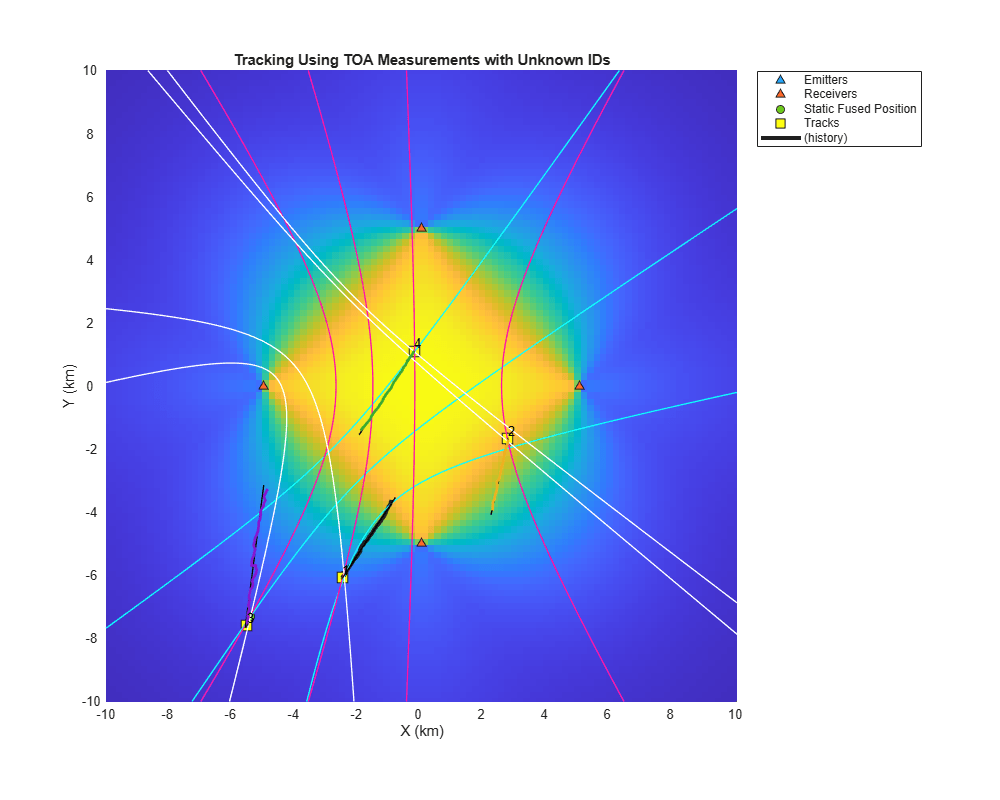Notice that the fuser algorithm is able to estimate the position of the object accurately most of the time. However, due to the presence of false alarms and missed measurements, the fusion algorithm may pick a wrong data association at some steps. This can lead to detections from ghost intersections. The tracker assists the static fusion algorithm by discarding these wrong associations as false alarms.

#### Tracking with Time-difference-of-Arrival (TDOA) Measurements

In this section, you configure the tracking algorithm for a system where TDOAs are formed from the receiver-pairs from multiple objects without any emitter identification. The calculation of TDOA from receiver pairs in a multi-object scenario may generate a few false alarms due to false peaks in the cross-correlation function. To simulate the TDOA measurements from such system, you increase the number of false alarms per receiver pair to 2.

The static fusion algorithm is configured similar to the previous section by using the `staticDetectionFuser` object. In contrast to the time-of-arrival fusion, here you use the measurement fusion function, `helperFuseTDOA`, to fuse multiple TDOAs into a fused measurement. You also use the helper function, `helperMeasureTDOA`, to define the transformation from fused measurement to TDOA measurement. As TDOA measurements do not contain or need information about the true signal emission time, you define the fused measurement as $\left[{\mathit{x}}_{\mathit{t}}\text{\hspace{0.17em}}{\mathit{y}}_{\mathit{t}\text{\hspace{0.17em}}}\text{\hspace{0.17em}}{\mathit{z}}_{\mathit{t}}\text{\hspace{0.17em}}\right]$. You set the `MaxNumSensors` to 3 as four receivers form three TDOA pairs.

```% Create scenario [scenario, rxPairs] = helperCreateMultiTargetTDOAScenario(4, 4); % Measurement statistics measNoise = 1e4; % 100 ns per receiver nFalse = 2; % 2 false alarms per receiver pair Pd = 0.95; % Detection probability per receiver pair % Release display release(display); display.Title = 'Tracking Using TDOA Measurements with Unknown IDs'; % Release tracker release(tracker); % Define fuser. tdoaFuser = staticDetectionFuser(MaxNumSensors=3,... MeasurementFormat='custom',... MeasurementFcn=@helperMeasureTDOA,... MeasurementFusionFcn=@helperTDOA2Pos,... DetectionProbability=Pd,... FalseAlarmRate=1e-8,... UseParallel=useParallel); while advance(scenario) % Current elapsed time time = scenario.SimulationTime; % Simulate TDOA detections with false alarms and missed detections tdoaDets = helperSimulateTDOA(scenario, rxPairs, measNoise, false, Pd, nFalse); % Fuse TDOA detections to esimate position detections of unidentified % and unknown number of emitters posDets = tdoaFuser(tdoaDets); % Update tracker with position detections if ~isempty(posDets) tracks = tracker(posDets, time); end % Update display display(scenario, receiverIDs, tdoaDets, posDets, tracks); end```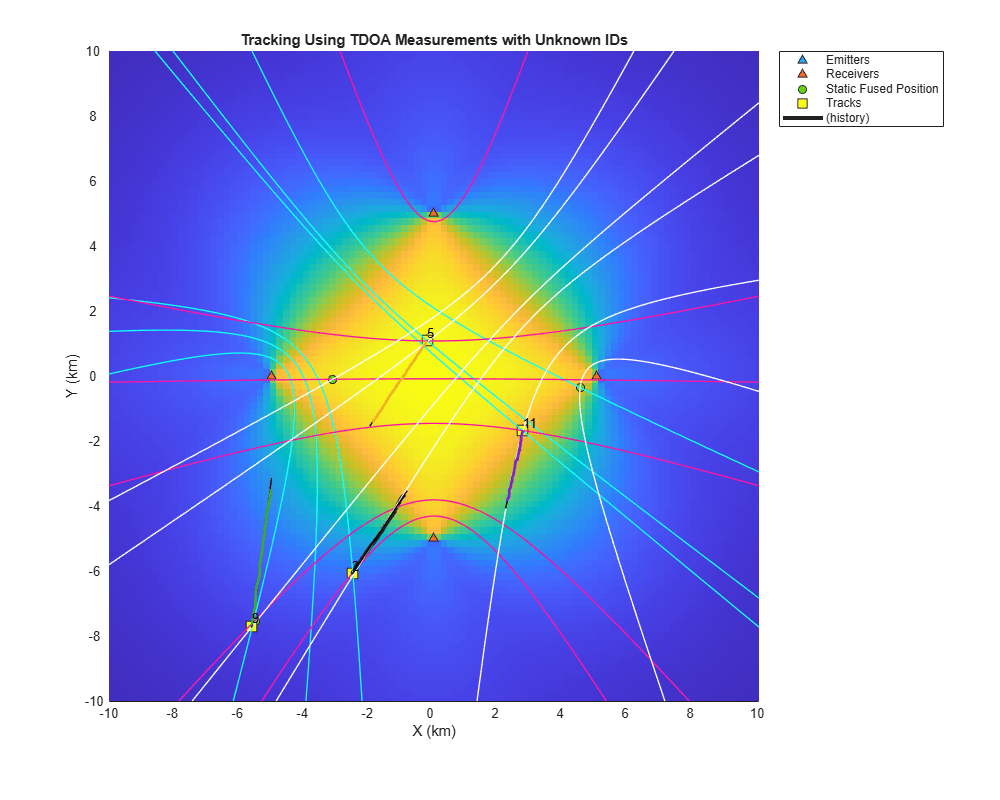With the defined measurement statistics in this example, the tracker maintains tracks on all the objects based on this network geometry. The geometry of the problem, the measurement accuracy, as well as the number of false alarms all have major impacts on the data association accuracy of the static fusion algorithm for both the TOA and TDOA systems with unknown data association. For the scenario used in this example, the static fusion algorithm was able to report true detections at sufficient time instants to maintain a track on true objects.

### Summary

In this example, you learned how to track single object as well as multiple objects using TDOA measurements. You learned about the challenges associated with multi-object tracking without emitter identification from the receivers and used a static fusion algorithm to compute data association at the measurement level.

### References

 Smith, Julius, and Jonathan Abel. "Closed-form least-squares source location estimation from range-difference measurements." IEEE Transactions on Acoustics, Speech, and Signal Processing 35.12 (1987): 1661-1669.

 Sathyan, T., A. Sinha, and T. Kirubarajan. "Passive geolocation and tracking of an unknown number of emitters." IEEE Transactions on Aerospace and Electronic Systems 42.2 (2006): 740-750.

### Supporting Functions

This section defines a few supporting functions used in this example. The complete list of helper functions can be found in the current working directory.

`helperTDOA2Pos`

```function varargout = helperTDOA2Pos(tdoaDets, reportDetection) % This function uses the spherical intersection algorithm to find the % object position from the TDOA detections assumed to be from the same % object. % % This function assumes that all TDOAs are measured with respect to the % same reference sensor. % % [pos, posCov] = helperTDOA2Pos(tdoaDets) returns the estimated position % and position uncertainty covariance. % % posDetection = helperTDOA2Pos(tdoaDets, true) returns the estimate % position and uncertainty covariance as an objectDetection object. if nargin < 2 reportDetection = false; end % Collect scaling information params = helperGetGlobalParameters; emissionSpeed = params.EmissionSpeed; timeScale = params.TimeScale; % Location of the reference receiver referenceLoc = tdoaDets{1}.MeasurementParameters(2).OriginPosition(:); % Formulate the problem. See  for more details d = zeros(numel(tdoaDets),1); delta = zeros(numel(tdoaDets),1); S = zeros(numel(tdoaDets),3); for i = 1:numel(tdoaDets) receiverLoc = tdoaDets{i}.MeasurementParameters(1).OriginPosition(:); d(i) = tdoaDets{i}.Measurement*emissionSpeed/timeScale; delta(i) = norm(receiverLoc - referenceLoc)^2 - d(i)^2; S(i,:) = receiverLoc - referenceLoc; end % Pseudo-inverse of S Swstar = pinv(S); % Assemble the quadratic range equation STS = (Swstar'*Swstar); a = 4 - 4*d'*STS*d; b = 4*d'*STS*delta; c = -delta'*STS*delta; Rs = zeros(2,1); % Imaginary solution, return a location outside coverage if b^2 < 4*a*c varargout{1} = 1e10*ones(3,1); varargout{2} = 1e10*eye(3); return; end % Two range values Rs(1) = (-b + sqrt(b^2 - 4*a*c))/(2*a); Rs(2) = (-b - sqrt(b^2 - 4*a*c))/(2*a); % If one is negative, use the positive solution if prod(Rs) < 0 Rs = Rs(Rs > 0); pos = 1/2*Swstar*(delta - 2*Rs(1)*d) + referenceLoc; else % Use range which minimize the error xs1 = 1/2*Swstar*(delta - 2*Rs(1)*d); xs2 = 1/2*Swstar*(delta - 2*Rs(2)*d); e1 = norm(delta - 2*Rs(1)*d - 2*S*xs1); e2 = norm(delta - 2*Rs(2)*d - 2*S*xs2); if e1 > e2 pos = xs2 + referenceLoc; else pos = xs1 + referenceLoc; end end % If required, compute the uncertainty in the position if nargout > 1 || reportDetection posCov = helperCalcPositionCovariance(pos,tdoaDets,timeScale,emissionSpeed); end if reportDetection varargout{1} = objectDetection(tdoaDets{1}.Time,pos,'MeasurementNoise',posCov); else varargout{1} = pos; if nargout > 1 varargout{2} = posCov; end end end function measCov = helperCalcPositionCovariance(pos,thisDetections,timeScale,emissionSpeed) n = numel(thisDetections); % Complete Jacobian from position to N TDOAs H = zeros(n,3); % Covariance of all TDOAs S = zeros(n,n); for i = 1:n e1 = pos - thisDetections{i}.MeasurementParameters(1).OriginPosition(:); e2 = pos - thisDetections{i}.MeasurementParameters(2).OriginPosition(:); Htdoar1 = (e1'/norm(e1))*timeScale/emissionSpeed; Htdoar2 = (e2'/norm(e2))*timeScale/emissionSpeed; H(i,:) = Htdoar1 - Htdoar2; S(i,i) = thisDetections{i}.MeasurementNoise; end Pinv = H'/S*H; % Z is not measured, use 1 as the covariance if Pinv(3,3) < eps Pinv(3,3) = 1; end % Insufficient information in TDOA if rank(Pinv) >= 3 measCov = eye(3)/Pinv; else measCov = inf(3); end % Replace inf with large number measCov(~isfinite(measCov)) = 100; % Return a true symmetric, positive definite matrix for covariance. measCov = (measCov + measCov')/2; end```

`helperInitHighSpeedKF`

```function filter = helperInitHighSpeedKF(detection) % This function initializes a constant velocity Kalman filter and sets a % higher initial state covariance on velocity components to account for % high speed of the object vehicles. filter = initcvkf(detection); filter.StateCovariance(2:2:end,2:2:end) = 500*eye(3); end```

`helperTOA2Pos`

```function [posTime, posTimeCov] = helperTOA2Pos(toaDetections) % This function computes the position and emission time of a target given % its time-of-arrival detections from multiple receivers. % % Convert TOAs to TDOAs for using spherical intersection [tdoaDetections, isValid] = helperTOA2TDOADetections(toaDetections); % At least 3 TOAs (2 TDOAs) required. Some TOA pairings can lead to an % invalid TDOA (> maximum TDOA). In those situations, discard the tuple by % using an arbitrary position with a large covariance. if numel(tdoaDetections) < 2 || any(~isValid) posTime = 1e10*ones(4,1); posTimeCov = 1e10*eye(4); else % Get position estimate using TDOA fusion. % Get time estimate using calculated position. if nargout > 1 % Only calculate covariance when requested to save time [pos, posCov] = helperTDOA2Pos(tdoaDetections); [time, timeCov] = helperCalcEmissionTime(toaDetections, pos, posCov); posTime = [pos;time]; posTimeCov = blkdiag(posCov,timeCov); else pos = helperTDOA2Pos(tdoaDetections); time = helperCalcEmissionTime(toaDetections, pos); posTime = [pos;time]; end end end```

helperCalcEmissionTime

```function [time, timeCov] = helperCalcEmissionTime(toaDetections, pos, posCov) % This function calculates the emission time of an object given its % position and obtained TOA detections. It also computes the uncertainty in % the estimate time. globalParams = helperGetGlobalParameters; emissionSpeed = globalParams.EmissionSpeed; timeScale = globalParams.TimeScale; n = numel(toaDetections); emissionTime = zeros(n,1); emissionTimeCov = zeros(n,1); for i = 1:numel(toaDetections) % Calculate range from this receiver p0 = toaDetections{i}.MeasurementParameters.OriginPosition(:); r = norm(pos - p0); emissionTime(i) = toaDetections{i}.Measurement - r/emissionSpeed*timeScale; if nargout > 1 rJac = (pos - p0)'/r; rCov = rJac*posCov*rJac'; emissionTimeCov(i) = rCov./emissionSpeed^2*timeScale^2; end end % Gaussian merge each time and covariance estimate time = mean(emissionTime); if nargout > 1 e = emissionTime - time; timeCov = mean(emissionTimeCov) + mean(e.^2); end end```

`helperMeasureTOA`

```function toa = helperMeasureTOA(posTime, params) % This function calculates the expected TOA at a receiver given an object % position and emission time and the receiver's measurement parameters % (OriginPosition) globalParams = helperGetGlobalParameters; emissionSpeed = globalParams.EmissionSpeed; timeScale = globalParams.TimeScale; r = norm(posTime(1:3) - params.OriginPosition); toa = posTime(4) + r/emissionSpeed*timeScale; end```

`helperMeasureTDOA`

```function toa = helperMeasureTDOA(pos, params) % This function calculates the expected TDOA measurement given object % position and measurement parameters of a TDOA detection globalParams = helperGetGlobalParameters; emissionSpeed = globalParams.EmissionSpeed; timeScale = globalParams.TimeScale; r1 = norm(pos(1:3) - params(1).OriginPosition); r2 = norm(pos(1:3) - params(2).OriginPosition); toa = (r1 - r2)/emissionSpeed*timeScale; end```